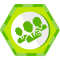## three.js 地形法向量生成 转

three.js 的PlaneGeometry 自带有法向量， 法向量分为两种 即 平面法向量 和 平面每个定点法向量。

材质编号_当前材质几何体组编号  作为几何体组的标识。

接着将相应的平面块 压入到对应的几何体组中。

控制每个几何体组的定点个数 小于 65535.

geometry.geometryGroups----->map形式访问几何体组

geometry.geometryGroupList-----> 数组形式访问几何体组

``````var pmat = new THREE.ShaderMaterial({
uniforms:{
light:{type:'v3', value:new THREE.Vector3()},
maxHeight:{type:'f', value:0},
minHeight:{type:'f', value:1},
},
attributes:{
displacement: {type:'f', value:[]},
vexNormal:{type:'v3', value:[]},
},

});``````

``````var v1 = new THREE.Vector3();
var v2 = new THREE.Vector3();

var distX = 2*3/(WIDTH-1);
var distY = 2*3/(HEIGHT-1);

var vexNormal = pmat.attributes.vexNormal.value;
var vertices = pmesh.geometry.vertices;

var lmat = new THREE.LineBasicMaterial({color:0xff0000});
for(var i = 0; i < vertices.length; i++)
{
var row = ~~(i/WIDTH);
var col = i%WIDTH;

var left = (col-1+WIDTH)%WIDTH;
var right = (col+1)%WIDTH;
var up = (row-1+HEIGHT)%HEIGHT;
var bottom = (row+1)%HEIGHT;

var l = value[row*WIDTH+left];
var r = value[row*WIDTH+right];
v1.set(distX, 0, r-l);

var u = value[up*WIDTH+col];
var b = value[bottom*WIDTH+col];
v2.set(0, distY, b-u);

v1.crossSelf(v2.clone()).normalize();

vexNormal.push(v1.clone());

var lgeo = new THREE.Geometry();
lgeo.vertices.push(new THREE.Vertex());
lgeo.vertices.push(new THREE.Vertex(v1.clone()));

var line = new THREE.Line(lgeo, lmat);
line.position.set(vertices[i].position.x, vertices[i].position.y, value[i]);

}``````

normalWorldMatrix 是 要的矩阵。

``````var pmat = new THREE.ShaderMaterial({
uniforms:{
light:{type:'v3', value:new THREE.Vector3()},
normalWorldMatrix:{type:'m4', value:new THREE.Matrix4()},
maxHeight:{type:'f', value:0},
minHeight:{type:'f', value:1},
},
attributes:{
displacement: {type:'f', value:[]},
vexNormal:{type:'v3', value:[]},
},
//wireframe:true,

});``````

normalWorldmatrix.value.getInverse(pmesh.matrixWorld).transpose();

nor = (normalWorldMatrix * vec4(vexNormal, 0)).xyz

lightDir = light-pos;

diffuse = max(dot(normalize(lightDir), nor), 0); 作为系数影响亮度。### liyong2### 评论(0)

three.js（六） 地形法向量生成

2012/09/18
254
0
three.js(八) bump map的生成

bump Map 主要用于增加表面的法向量细节。例如一个平面其法向量处处相同，即使使用了纹理，光照下的表现仍然不够真实。这时可以扰动表面面片的方向量，从而形成比较真实的光照效果。 类似于地...

2012/09/18
610
0
Threejs创建三维文字TextGeometry

THREE.js 封装了 TextGeometry 类可以很容易地生成三维文字 TextGeometry(text : String, parameters : Object) 参数说明 text — The text that needs to be shown. （要显示的字符串）par...

osc_y8w65yuq
01/14
1
0
three.js 04-04 之 MeshNormalMaterial 材质

zhulx_sz
04/01
0
0

[toc] 1. 原理 以前一直以为对DEM的渲染就是简单的根据DEM的高度不同赋予不同的颜色就可以。后来实际这么做的时候获取的效果跟别的软件相比，根本体现不出地形起伏的变化。如果要体现出地形的...

osc_tzh2wzwm
04/16
2
0

Windows 10 中安装 Anaconda 3

honeymoose
24分钟前
19
0

24分钟前
25
0

2.简介： E聊SDK是一套适用于PC端, 移动端的即时通讯解决方案，源代码开放。E聊整合了即时通讯的基础能力，使用E聊，您可以让您的应用快速接入即时聊天的功能。E聊现已适配PC Web, 移动Web, ...

E聊
37分钟前
9
0

40分钟前
22
0
403禁止vs 401未经授权的HTTP响应 - 403 Forbidden vs 401 Unauthorized HTTP responses

fyin1314
55分钟前
19
0